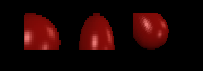# TextureCoordinate

## Introduction

The TextureCoordinate property provides the ability for Sprites to display part of a Texture2D. By default, Sprites display their entire Texture. This behavior is desirable in many situations, but you may want to display only part of an image if you are using sprite sheets or in certain graphical effects. TextureCoordinates also let you display “more” of a texture. Examples include stretching the edge pixels or wrapping (tiling) the texture.

## Understanding TextureCoordinates and Pixels

By default all texture coordinates for a Sprite are either 0 or 1. This might seem a little weird considering Sprites usually do not reference textures of only 1 pixel size. Furthermore, regardless of the size of the referenced texture the texture coordinates will always default to 0 or 1. What does this mean?

For a number of reasons, 3D APIs like DirectX and XNA use 0 – 1 for texture coordinates regardless of the width of the texture. The coordinate (0,0) indicates the top-left of the texture while the coordinate (1,1) represents the bottom right of the coordinate. The coordinate (1,0) is the top right.

Therefore, if you are used to working with pixels, you need to divide your desired pixel coordinate by the width and height of your texture to get the desired texture coordinate.

The following code converts a pixel coordinate to a texture coordinate.

```int desiredPixelCoordinateX = 16;
int desiredPixelCoordinateY = 22;
```
```float textureCoordinateX = desiredPixelCoordinateX / (float)someTexture.Width;
float textureCoordinateY = desiredPixelCoordinateY / (float)someTexture.Height;
```

## Texture Coordinates

Sprites support setting texture coordinates on each vertex. This is useful for drawing from a sprite sheet. The vertex at index 0 is the top left. The count increases clockwise as follows:

```0----1
|    |
3----2
```

The default values are:

```mVertices.TextureCoordinate.X = 0;
mVertices.TextureCoordinate.Y = 0;
```
```mVertices.TextureCoordinate.X = 1;
mVertices.TextureCoordinate.Y = 0;
```
```mVertices.TextureCoordinate.X = 1;
mVertices.TextureCoordinate.Y = 1;
```
```mVertices.TextureCoordinate.X = 0;
mVertices.TextureCoordinate.Y = 1;
```

Or graphically:

```(0,0)----------(1, 0)
|                   |
|                   |
|                   |
|                   |
|                   |
(0,1)----------(1, 1)
```

Values range between 0 and 1 regardless of texture size, so conversions are necessary to work in pixel values.

The following code creates three Sprites with modified texture coordinates.

```Sprite sprite1 = SpriteManager.AddSprite("redball.bmp");
sprite1.Vertices.TextureCoordinate.X = .4f;
sprite1.Vertices.TextureCoordinate.Y = .6f;
sprite1.Vertices.TextureCoordinate.X = .4f;
sprite1.Vertices.TextureCoordinate.Y = .6f;
```
```Sprite sprite2 = SpriteManager.AddSprite("redball.bmp");
sprite2.Vertices.TextureCoordinate.Y = .5f;
sprite2.Vertices.TextureCoordinate.Y = .5f;
sprite2.X = 3;
```
```// This Sprite's texture coordinates are not axis aligned.
// This can cause graphical artifacts so be careful.
sprite3.Vertices.TextureCoordinate.X = .2f;
sprite3.Vertices.TextureCoordinate.Y = .3f;
sprite3.X = 6;
```## TextureCoordinate Properties

Rather than working directly with the Vertices property, the Sprite exposes four properties to help set texture coordinates. These can be used to set texture coordinates much easier. The following two pieces of code produce identical results:

```sprite1.Vertices.TextureCoordinate.X = .4f;
sprite1.Vertices.TextureCoordinate.Y = .6f;
sprite1.Vertices.TextureCoordinate.X = .4f;
sprite1.Vertices.TextureCoordinate.Y = .6f;
```
```// AND
```
```sprite1.LeftTextureCoordinate = .4f;
sprite1.BottomTextureCoordinate = .6f;
```

## Tiling Graphics

Using TextureCoordinates outside of the 0-1 range enables you to tile textures. In other words, to tile an object vertically 5 times, you would want to set the right side TextureCoordinate’s X’s to 5:

```mVertices.TextureCoordinate.X = 5;
mVertices.TextureCoordinate.X = 5;
```